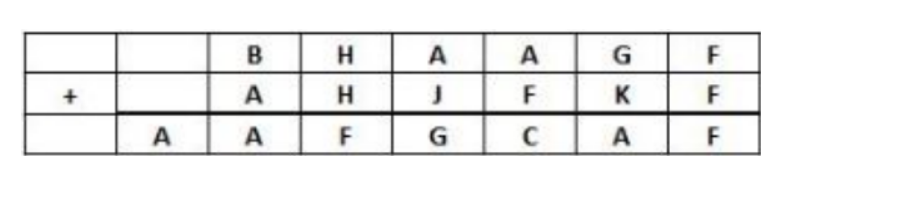# The following table represents addition of two | CAT Logical Reasoning

## CAT 2019 - Slot 1 - Set 7 - The following table represents addition of two

##### The following table represents addition of two six-digit numbers given in the first and the second rows, while the sum is given in the third row. In the representation, each of the digits 0, 1, 2, 3, 4, 5, 6, 7, 8, 9 has been coded with one letter among A, B, C, D, E, F, G,H, J, K, with distinct letters representing distinct digits.Q. 1: Which digit does the letter A represent?

1. Explanation: As we can see that F + F gives F unit digit it is possible only when F = 0 So F has to be 0.
Now H + H + carry (if it is there) gives F as unit digit it is possible when H = 5 (we have max 9+9 = 18 so we will never have a carry equal to 2 so H + H = 10 is only possibility) Thus H has to be 5. A also is necessarily 1 because maximum carryover can be 1. C will have to be 2 because 1 is already taken now. And only number possible for B is 9 then to get 1 below. Thus we get:
9 5 1 1 G 0
1 5 J 0 K 0
1   1 0 G 2 1 0
Now possible J, G, K are 3 ,4, 7 or 6,7,4 or 7,8,3

Q. 2: Which digit does the letter B represent?

Q. 3: Which among the digits 3, 4, 6 and 7 cannot be represented by the letter D?

Correct Answer: D can never be 7 because J,G or K are always 7

Q. 4: Which among the digits 4, 6, 7 and 8 cannot be represented by the letter G?

Correct Answer: G cannot be represented by 6

### Past Year Question Paper & SolutionsCounselling Session
By IIM Mentor

### Free Material Area